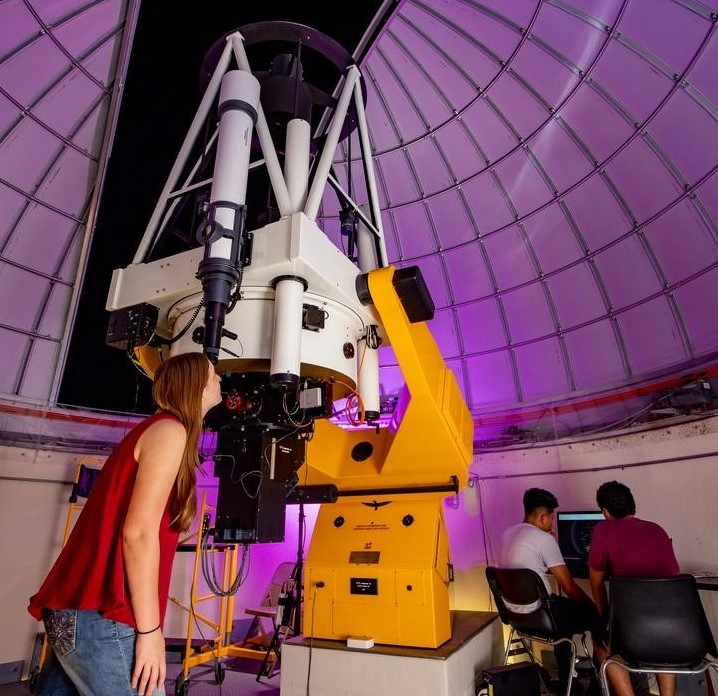## Aerospace, Physics, and Space Science Faculty Publications

Article

#### Publication Title

Journal of High Energy Physics

#### Abstract

A measurement of inclusive W and Z production cross sections in pp collisions at √s = 7 TeV is presented. The electron and muon decay channels are analyzed in a data sample collected with the CMS detector at the LHC and corresponding to an integrated luminosity of 36 pb−1. The measured inclusive cross sections are σ(pp → WX) ×  B(W→ℓν) = 10.31 ± 0.02 (stat.) ± 0.09 (syst.) ± 0.10 (th.) ± 0.41 (lumi.) nb and σ(pp → ZX) ×  B(Z→ℓ+ℓ−) = 0.974 ± 0.007 (stat.) ± 0.007 (syst.) ± 0.018 (th.) ± 0.039 (lumi.) nb, limited to the dilepton invariant mass range 60 to 120 GeV. The luminosity-independent cross section ratios are (σ(pp → WX) ×  B(W→ℓν)) / (σ(pp → ZX) ×  B(Z→ℓ+ℓ−)) = 10.54 ± 0.07 (stat.) ± 0.08 (syst.) ± 0.16 (th.) and (σ(pp → W+X) ×  B(W+→ℓ+ν)) / (σ(pp → W−X) ×  B(W−→ℓ−ν¯)) = 1.421 ± 0.006 (stat.) ± 0.014 (syst.) ± 0.029 (th.). The measured values agree with next-to-next-to-leading order QCD cross section calculations based on recent parton distribution functions.

#### DOI

10.1007/JHEP10(2011)132

2011

COinS